Mathematical and Physical Journal
for High Schools
Issued by the MATFUND Foundation
 Already signed up? New to KöMaL?

# KöMaL Problems in Physics, November 2008

Show/hide problems of signs:## Problems with sign 'M'

Deadline expired on December 11, 2008.

M. 291. Measure the resistance of a 40 W tungsteen filament lamp at different temperatures. (Between -15 oC and +40 oC.) Determine the temperature coefficient of the resistance. It is advisable to use a digital ohmmeter. Do not use the mains power (220 V AC).

(6 pont)

statistics## Problems with sign 'P'

Deadline expired on December 11, 2008.

P. 4104. 1 kg broken ice is placed into a 2-l container, which has rigid walls, and then the container is sealed hermetically. While the temperature remains 0 oC the total amount of ice melts. By what percent does the pessure of the air enclosed in the container change?

(3 pont)

statistics

P. 4105. Water and oil of equal mass can be poured into a square-based prism. In which case will the force exerted on the vertical faces of the prism be greater?

(4 pont)

statistics

P. 4106. A biro (ballpoint pen), which contains a spring, is pressed against a support at the bottom of an inclined plane as shown in the figure, and then suddenly it is released. The table below shows our measured data, the distance covered by the ballpoint pen as a function of the angle of inclination of the plane: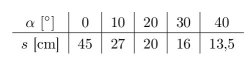Using this table determine the initial speed of the biro and the coefficient of kinetic friction between the plane and the biro.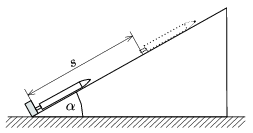(4 pont)

statistics

P. 4107. A small beetle of mass m is slowly crawling out from the bottom of a hemi-sphere shaped shell. The mass of the shell is M and its radius is R. How much work does the beetle perform until it reaches the rim of the hemi-sphere? (The coefficient of static friction between the beetle and the shell is large enough.)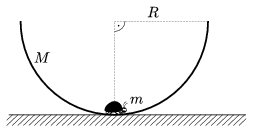(4 pont)

statistics

P. 4108. An ice-hockey player hits the puck with an initial speed of v0 towards the board fence. The mass of the puck is m and its radius is R. The puck is not rotating and the angle between the velocity and the board fence is. The component of the velocity of the puck which is perpendicular to the board fence changes by a factor of k (k<1). What will the rotational energy of the puck be after the collision with the board fence? The coefficient of kinetic friction between the board fence and the puck is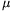and the friction on the ice is negligible.

(5 pont)

statistics

P. 4109. Using a bubble blower a lot of bubles of different sizes can be blown. These bubbles descend slowly. Assuming that all bubbles have the same thickness wall, which bubbles descend more quickly, the ones which have greater radius or the ones which have smaller radius?

(4 pont)

statistics

P. 4110. The horizontal part of the U-shaped tube shown in the figure is 10 cm, and its vertical branches are long enough. The cross section of the tube is 1 cm2. First, 20 cm3 water is poured into the tube, then 20 cm3 oil is poured into the left branch.,. The whole system is accelerated towards the left, at an acceleration of a. Determine and graph the difference between the level of water and the level of oil as a function of the acceleration.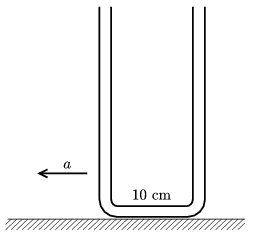(5 pont)

statistics

P. 4111. The wheel of a tractor is moving at a velocity of v. The diameter of the wheel is D. From any point of the rim of the wheel pieces of mud can flake off. What is the longest time during which a piece of mud may reach the ground? At what position does the piece of mud which falls in the longest time is released from the wheel?

(5 pont)

statistics

P. 4112. A particle of charge Q and of negligible initial speed is accelerated through a potential difference of U. The particle reaches a region of uniform magnetic field of induction B, where it undergoes circular motion. What is the magnetic momentum of the circular current''?

(4 pont)

statistics

P. 4113. A spherical cap of radius r and of width d, is placed onto the top of the sphere of the Van de Graaff generator, the radius of which is R. The spherical cap is made of aluminum foil of density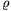. At what voltage value will the spherical cap ascend from the sphere of the Van de Graaff generator?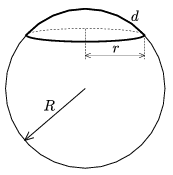(5 pont)

statistics